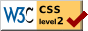# Editors and Cooperating editors of the Psychological Bulletin,  1904-1913

This table was constructed from the title pages of the Psychological Bulletin for its first 10 years. Please note that J. B. Watson appears in both the list of Co-operating Editors (1906-1908) and of Editors (1909-1913). The color version highlights editors from the two schools that dominated the journal during the period. Editors in pink were affiliated with Baldwin's institution,—Johns Hopkins (25 years of combined service); those affiliated with the University of Chicago were highlighted in blue (22 years of combined service).

Editors of the Psychological Bulletin
1900s1910s
Editor Affiliation 4567890123
J. Mark Baldwin Johns Hopkins University x x x x x x
Howard C. Warren Princeton University x x x x x x x x x x
Charles H. Judd Yale University x x x x x
Arthur Peirce Smith College             x x x x
J. B. Watson Johns Hopkins University           x x x x x
James R. Angell University of Chicago           x x x x x

Co-operating Editors
J. W. Baird University of Illinois     x x x x x x x x
H. Heath Bawden Vassar,  Cincinatti x x x x x
Madison Bentley University of Illinois             x x x x
T. L. Bolton University of Nebraska x x x x x x
E. F. Buchner Alabama, Johns Hopkins x x x x x x x x x x
H. A. Carr University of Chicago             x x x x
R. Dodge Wesleyan University x x x x x x
Knight Dunlap Johns Hopkins University             x x x x
S. Franz Gov. Hosp. for the Insane           x
J. G. Hibben Princeton University x x x x x
Irving King Pratt Institute, University of Iowa x x x x x x
E. B. Holt Harvard University             x x x x
J. H. Leuba Bryn Mawr College x x x x x x x x x x
R. MacDougall New York University x x x x x x x x x x
C. N. MacAllister Yale University x x
A. Meyer N. Y. State Pathol. Institute x x x x x
Max Meyer University of Missouri x x x x x x x x x x
G. H. Mead University of Chicago               x x x
R. M. Ogden University of Tennessee           x x x x x
I. W. Riley Vassar College x x x x x x
W. D. Scott Northwestern University             x x x x
C. E. Seashore University of Iowa           x
G. M. Stratton Johns Hopkins University x x x x x
E. J. Swift Washington University       x x x x x x x
G. A. Tawney Beloit College x x x x
J. H. Tufts University of Chicago x x x x x x x
W. M. Urban Trinity College x x x x x
M. F. Washburn Vassar College           x x x x x
J. B. Watson University of Chicago     x x x
W. M. Wheeler Am. Mus. Natural History x x   x x
R. S. Woodworth Columbia University             x x x x

### Notes

No notes